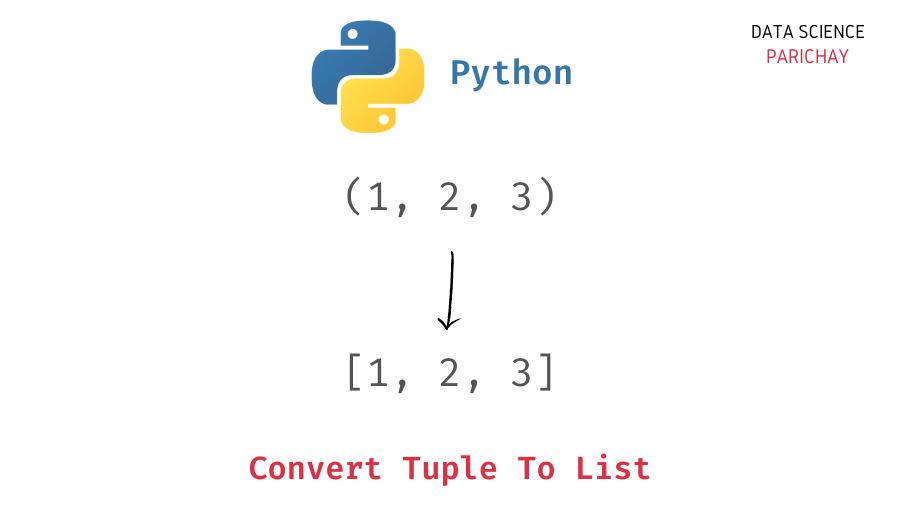# Convert Tuple to a List in Python – With Examples

In this tutorial, we will look at how to convert a tuple to a list in Python with the help of some examples.

Both tuples and lists are used to store ordered collection of objects but lists and tuples are not the same. Lists are mutable whereas tuples, on the other hand, are immutable. That is, elements in a tuple cannot be altered after it’s created. Thus, the conversion from tuple to list is a common use-case in Python.

You can use the Python built-in `list()` function to convert a tuple to a list. Alternatively, you can also use a list comprehension to create list with elements from the tuple. The following is the syntax.

```# create list from tuple t
# using list() function
ls = list(t)
# using list comprehension
ls = [item for item in t]```

Let’s now look at some examples of using the above methods to create list from a tuple.

The built-in Python `list()` function is used to create a list. Pass the tuple as an argument to the `list()` function to create a list with the tuple elements.

```# create a tuple
t = (1, 2, 3, "cat")
# create list from tuple
ls = list(t)
print(ls)```

Output:

`[1, 2, 3, 'cat']`

We get the resulting list containing the elements from the tuple.

You can also create a list from tuple elements using a list comprehension. Here, we iterate through the elements of the tuple in a list comprehension and create a list of tuple elements.

📚 Data Science Programs By Skill Level

Introductory

Intermediate ⭐⭐⭐

🔎 Find Data Science Programs 👨‍💻 111,889 already enrolled

Disclaimer: Data Science Parichay is reader supported. When you purchase a course through a link on this site, we may earn a small commission at no additional cost to you. Earned commissions help support this website and its team of writers.

```# create a tuple
t = (1, 2, 3, "cat")
# create list from tuple
ls = [item for item in t]
print(ls)```

Output:

`[1, 2, 3, 'cat']`

We get the same result as above.

In this tutorial, we looked at two methods to convert a tuple to a list in Python. Both the methods are straightforward. Using the `list()` function is direct and easy whereas a list comprehension can be handy when you want to perform some additional operations on the tuple elements. For example, creating a list of squares from the tuple elements.

You might also be interested in –

•